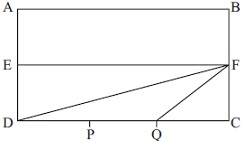# User ForumSubject :IMO    Class : Class 8

The area of the given rectangle ABCD is 180 cm2. EF divides the rectangle into two equal parts. If DP = PQ = QC, find the ratio of the area of triangle DFQ to the area of triangle DEF.A3 : 4
B1 : 2
C2 : 3
D1 : 3

Answer is (C) 2:3 some technical glitch this is